# Math in Focus Grade 4 Chapter 12 Practice 3 Answer Key Rectangles and Squares

This handy Math in Focus Grade 4 Workbook Answer Key Chapter 12 Practice 3 Rectangles and Squares detailed solutions for the textbook questions.

## Math in Focus Grade 4 Chapter 12 Practice 3 Answer Key Rectangles and Squares

Find the area of each figure.

Example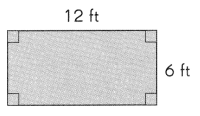Area of the rectangle = 12 × 6
= 72 ft2
The area of the rectangle is 72 square feet.

Question 1.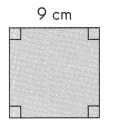Area of the square = _________ × __________
= __________ cm2
The area of the square is _____________ square centimeters.
Area of the square = 9 cm × 9 cm
= 81 cm2
The area of the square is 81 square centimeters.

Example

The area of a rectangular hall is 78 square yards. Its width is 6 yards. Find the length.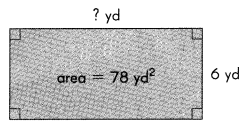Length × width = area
Length × 6 = 78 yd2
Length = 78 ÷ 6
= 13 yd
The length of the hall is 13 yards.

Question 2.
A rectangle has an area of 56 square centimeters. Its length is 8 centimeters. Find the width.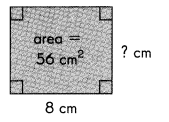The width of the rectangle is ___________ centimeters.
Length = 8 cm
width = ?
Area of the rectangle = 56 cm2
Area of the rectangle = length × width
56 cm2 = 8 cm x width
56 cm÷ 8 cm = width
7 cm = width
The width of the rectangle is 7 centimeters.

Question 3.
The area of a rectangular carpet is 84 square meters. Its width is 7 meters.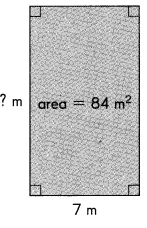a. Find the length.
Length = ?
width = 7 m
Area of the rectangular carpet = 84 m2
Area of the rectangular carpet = length × width
84 m2 = length x 7 m
84 m÷ 7 m = length
12 m = length
The length of the rectangular carpet is 12 meters.

b. Find the perimeter of the carpet.
Perimeter of the carpet = 7 m + 12 m + 7 m + 12 m
= 38 m
Perimeter of the carpet is 38 meters.

Question 4.
The area of a square is 64 square inches. Find the length of one side of the square.
(Hint: What number multiplied by itself is equal to 64?)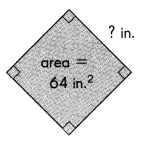Area = side × side = s2
Where s is the length of the side of the square.
Area of a square is 64 square inches.
64 in2= s2
(8 in)2= s2
8 inches = s
The length of one side of the square is 8 inches.

Question 5.
The area of a square garden is 100 square meters.
a. Find the length of each side of the garden.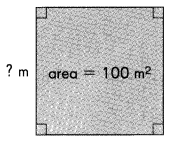A square is a regular polygon with four sides.
Area = width × height
But since the width and height are by definition the same, the formula is usually written as
Area = side × side = s2
where s is the length of one side.
To find the length of each side of the garden we know that the area is 100applying the area formula and solving for s, the length of one side, we get that
100 m2= s2
(10 m)2 = s2
10 m = s
The length of each side of the garden is 10 m.

b. Find the perimeter of the garden.
In the case of a square, all four sides are the same length, so the perimeter is four times the length of a side.
We find that the length of each side is 10 m. Therefore, the perimeter is
Perimeter = 4 × s
= 4 x 10 m
= 40 m
where s is the length of any one side.
The perimeter of the garden is 40 m.

Question 6.
The area of a rectangular recreation area is 45 square miles. Its width is 5 miles.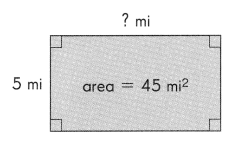a. Find the length.
Length = ?
width = 5 mi
Area of the rectangular recreation = 45 mi2
Area of the rectangular recreation = length × width
45 mi2 = length x 5 mi
45 mi÷ 5 mi = length
9 mi = length
The length of the rectangular recreation is 9 miles.

b. Find the perimeter.
Perimeter of the rectangular recreation = 9 mi + 5 mi + 9 mi + 5 mi
= 28 miles
Perimeter of the rectangular recreation is 28 miles.

Question 7.
The perimeter of a rectangular poster is 156 inches. Its width is 36 inches.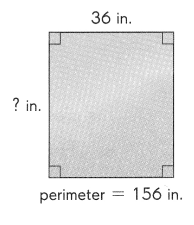a. Find the length.
Length = ?
width = 36 in
perimeter  of a rectangular poster = 156 in
Perimeter of the rectangular poster = length + width + length + width
156 in = l + 36 in + l + 36 in
156 in = 2l + 72 in
156 in – 72 in = 2l
84 in = 2l
42 in = l
The length of the rectangular poster is 42 inches.

b. Find the area.